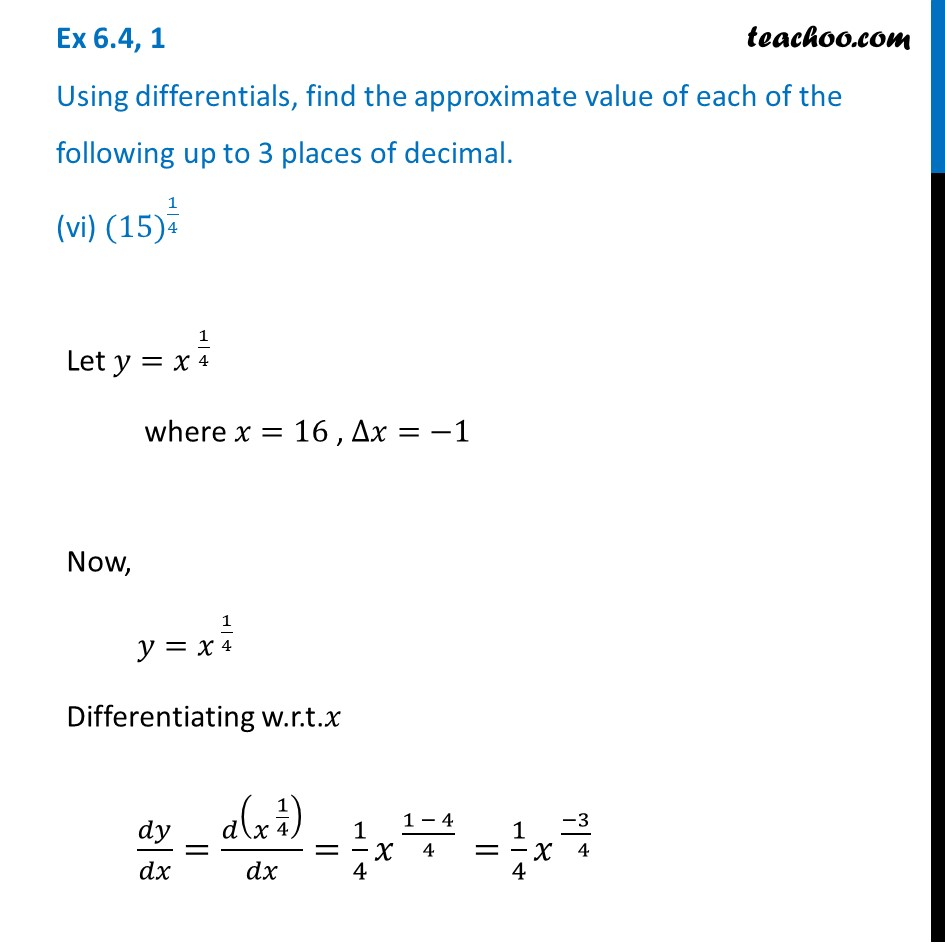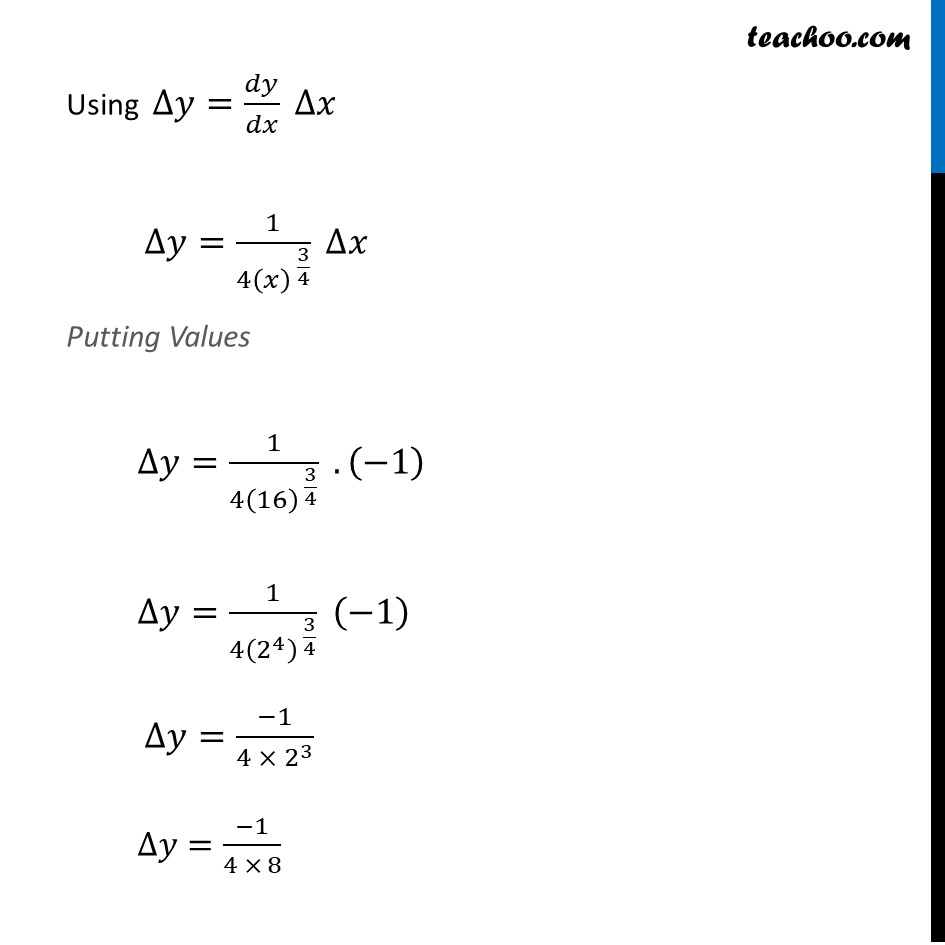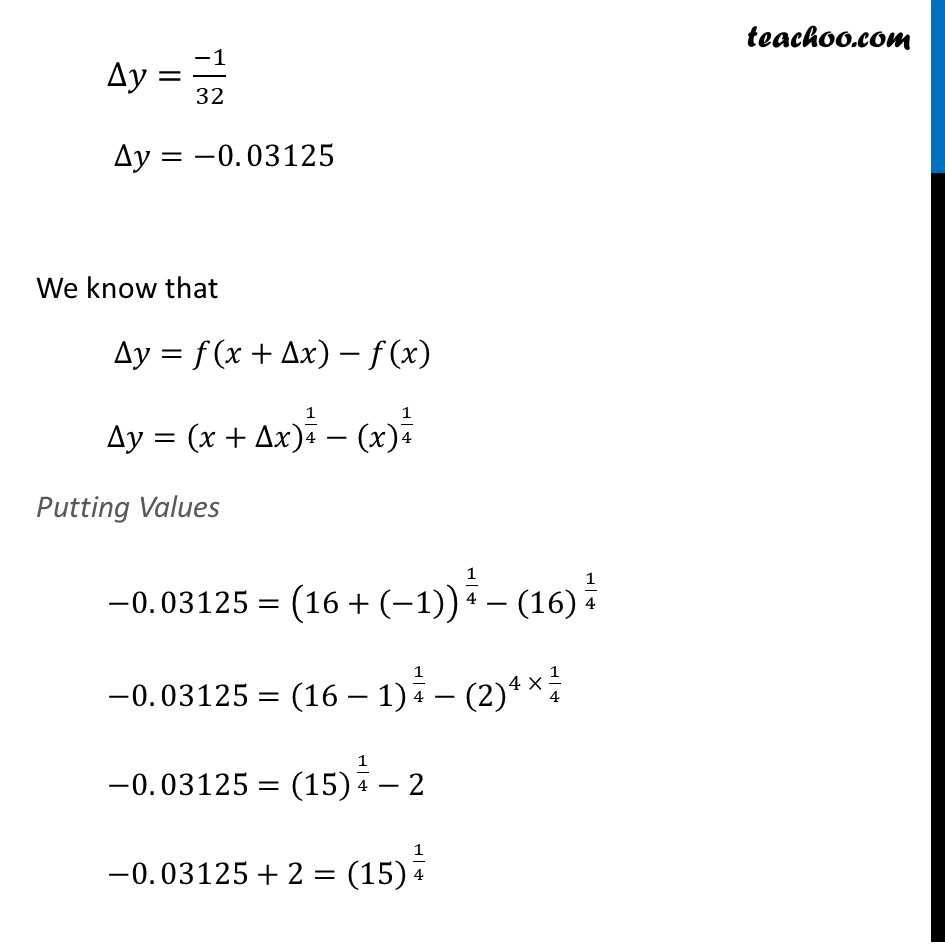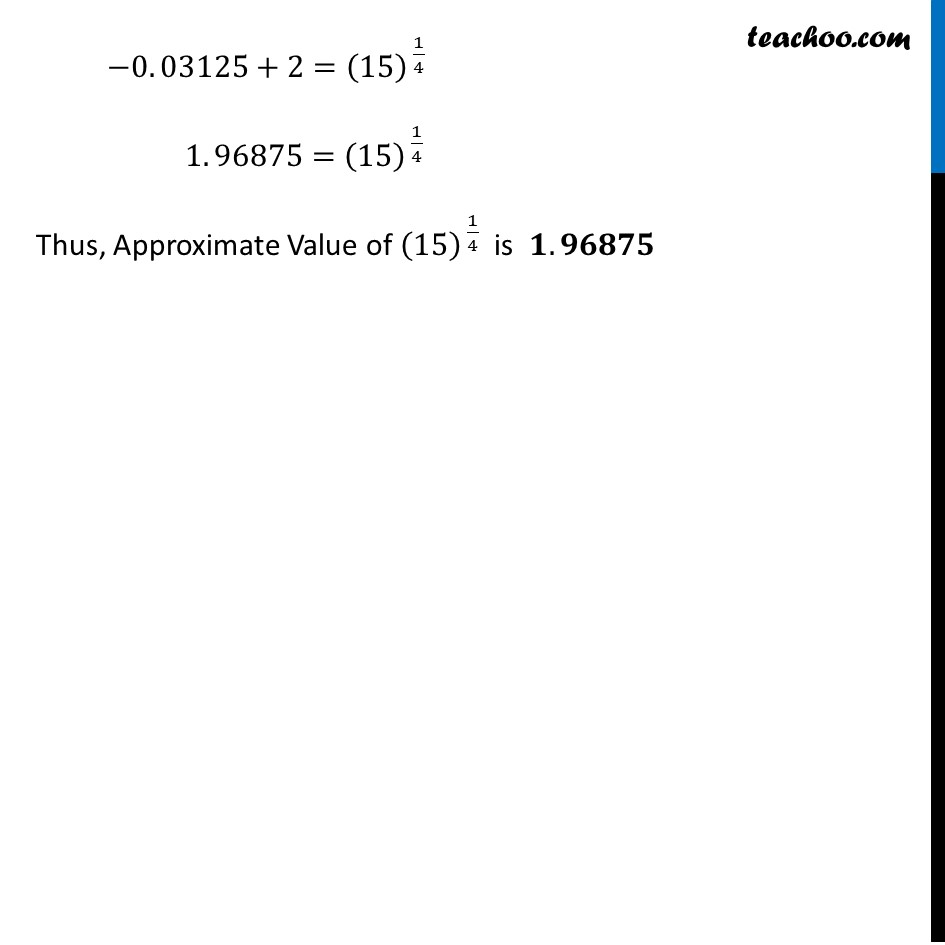Approximations (using Differentiation)

Chapter 6 Class 12 Application of Derivatives
Serial order wiseLearn in your speed, with individual attention - Teachoo Maths 1-on-1 Class

### Transcript

Question 1 Using differentials, find the approximate value of each of the following up to 3 places of decimal. (vi) 〖(15)〗^(1/4)Let 𝑦=〖𝑥 〗^(1/4) where 𝑥=16 , ∆𝑥=−1 Now, 𝑦=𝑥^( 1/4) Differentiating w.r.t.𝑥 𝑑𝑦/𝑑𝑥=𝑑(𝑥^( 1/4) )/𝑑𝑥=1/4 𝑥^( (1 − 4)/4 )=1/4 𝑥^( (−3)/( 4) ) Using ∆𝑦=𝑑𝑦/𝑑𝑥 ∆𝑥 ∆𝑦=1/(4(𝑥)^( 3/4) ) ∆𝑥 Putting Values ∆𝑦=1/(4(16)^( 3/4) ) . (−1) ∆𝑦=1/(4(2^4 )^( 3/4) ) (−1) ∆𝑦=(−1)/(4 × 2^3 ) ∆𝑦=(−1)/(4 × 8) ∆𝑦=(−1)/32 ∆𝑦=−0. 03125 We know that ∆𝑦=𝑓(𝑥+∆𝑥)−𝑓(𝑥) ∆𝑦=(𝑥+∆𝑥)^(1/4)−(𝑥)^(1/4) Putting Values −0. 03125=(16+(−1))^( 1/4)−〖(16) 〗^(1/4) −0. 03125=(16−1)^( 1/4)−(2)^(4 × 1/4) −0. 03125=(15)^( 1/4)−2 −0. 03125+2=(15)^( 1/4) −0. 03125+2=(15)^( 1/4) 1. 96875=(15)^( 1/4) Thus, Approximate Value of (15)^( 1/4) is 𝟏. 𝟗𝟔𝟖𝟕𝟓